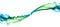# Curry and Function CompositionSmoke Art Cubes to Smoke — MattysFlicks — (CC BY 2.0)

# What is a curried function?

`// add = a => b => Numberconst add = a => b => a + b;`
`const result = add(2)(3); // => 5`

# What is point-free style?

`function foo (/* parameters are declared here*/) {  // ...}const foo = (/* parameters are declared here */) => // ...const foo = function (/* parameters are declared here */) {  // ...}`
`// inc = n => Number// Adds 1 to any number.const inc = add(1);inc(3); // => 4`
`const inc10 = add(10);const inc20 = add(20);inc10(3); // => 13inc20(3); // => 23`
`inc(3) // 4`

# Why do we curry?

`g: a -> bf: b -> c`
`// Algebra definition, borrowing the `.` composition operator// from Haskellh: a -> ch = f . g = f(g(x))`
`const g = n => n + 1;const f = n => n * 2;const h = x => f(g(x));h(20); //=> 42`
`f . g = f(g(x))`
`const compose = (f, g) => x => f(g(x));`
`f . g . h`
`const compose = (...fns) => x => fns.reduceRight((y, f) => f(y), x);`
`const g = n => n + 1;const f = n => n * 2;// replace `x => f(g(x))` with `compose(f, g)`const h = compose(f, g);h(20); //=> 42`

# Trace

`const trace = label => value => {  console.log(`\${ label }: \${ value }`);  return value;};`
`const compose = (...fns) => x => fns.reduceRight((y, f) => f(y), x);const trace = label => value => {  console.log(`\${ label }: \${ value }`);  return value;};const g = n => n + 1;const f = n => n * 2;/*Note: function application order isbottom-to-top:*/const h = compose(  trace('after f'),  f,  trace('after g'),  g);h(20);/*after g: 21after f: 42*/`
`const pipe = (...fns) => x => fns.reduce((y, f) => f(y), x);`
`const pipe = (...fns) => x => fns.reduce((y, f) => f(y), x);const trace = label => value => {  console.log(`\${ label }: \${ value }`);  return value;};const g = n => n + 1;const f = n => n * 2;/*Now the function application orderruns top-to-bottom:*/const h = pipe(  g,  trace('after g'),  f,  trace('after f'),);h(20);/*after g: 21after f: 42*/`

# Curry and Function Composition, Together

`const map = fn => mappable => mappable.map(fn);const pipe = (...fns) => x => fns.reduce((y, f) => f(y), x);const log = (...args) => console.log(...args);const arr = [1, 2, 3, 4];const isEven = n => n % 2 === 0;const stripe = n => isEven(n) ? 'dark' : 'light';const stripeAll = map(stripe);const striped = stripeAll(arr); log(striped);// => ["light", "dark", "light", "dark"]const double = n => n * 2;const doubleAll = map(double);const doubled = doubleAll(arr);log(doubled);// => [2, 4, 6, 8]`
`f: a => bg:      b => ch: a    =>   c`
`f: a => bg:     (x, b) => ch: a    =>   c`
`const pipe = (...fns) => x => fns.reduce((y, f) => f(y), x);const trace = label => value => {  console.log(`\${ label }: \${ value }`);  return value;};const g = n => n + 1;const f = n => n * 2;const h = pipe(  g,  trace('after g'),  f,  trace('after f'),);h(20);/*after g: 21after f: 42*/`
`const pipe = (...fns) => x => fns.reduce((y, f) => f(y), x);const trace = (label, value) => {  console.log(`\${ label }: \${ value }`);  return value;};const g = n => n + 1;const f = n => n * 2;const h = pipe(  g,  // the trace() calls are no longer point-free,  // introducing the intermediary variable, `x`.  x => trace('after g', x),  f,  x => trace('after f', x),);h(20);`
`const pipe = (...fns) => x => fns.reduce((y, f) => f(y), x);const trace = value => label => {  console.log(`\${ label }: \${ value }`);  return value;};const g = n => n + 1;const f = n => n * 2;const h = pipe(  g,  // the trace() calls can't be point-free,  // because arguments are expected in the wrong order.  x => trace(x)('after g'),  f,  x => trace(x)('after f'),);h(20);`
`const flip = fn => a => b => fn(b)(a);`
`const flippedTrace = flip(trace);`
`const flip = fn => a => b => fn(b)(a);const pipe = (...fns) => x => fns.reduce((y, f) => f(y), x);const trace = value => label => {  console.log(`\${ label }: \${ value }`);  return value;};const flippedTrace = flip(trace);const g = n => n + 1;const f = n => n * 2;const h = pipe(  g,  flippedTrace('after g'),  f,  flippedTrace('after f'),);h(20);`
`const trace = label => value => {  console.log(`\${ label }: \${ value }`);  return value;};`
`const trace = label => value => {  console.log(`\${ label }: \${ value }`);  return value;};const traceAfterG = trace('after g');`
`const traceAfterG = value => {  const label = 'after g';  console.log(`\${ label }: \${ value }`);  return value;};`
`const pipe = (...fns) => x => fns.reduce((y, f) => f(y), x);const trace = label => value => {  console.log(`\${ label }: \${ value }`);  return value;};// The curried version of trace()// saves us from writing all this code...const traceAfterG = value => {  const label = 'after g';  console.log(`\${ label }: \${ value }`);  return value;};const g = n => n + 1;const f = n => n * 2;const h = pipe(  g,  traceAfterG,  f,  trace('after f'),);h(20);`

# Conclusion

Written by

Written by

## Eric Elliott

#### Make some magic. #JavaScript